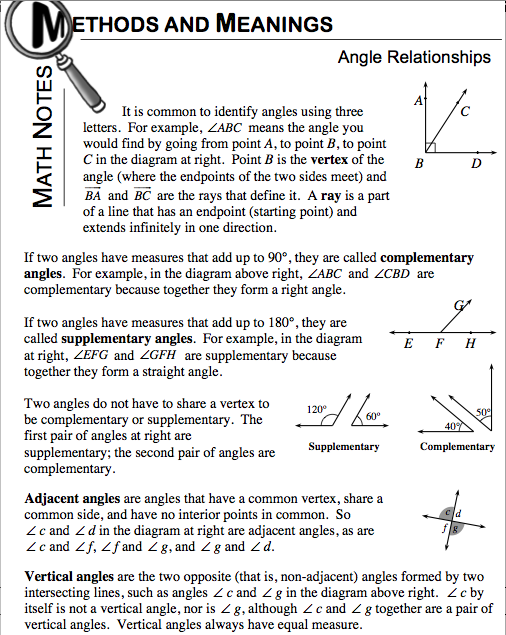### Home > CCAA > Chapter 9 Unit 8 > Lesson CC2: 9.1.1 > Problem9-21

9-21.

Review the definitions of complementary, supplementary, and vertical angles. Use the information to answer the following questions.  Homework Help ✎

1. Two angles are complementary. If one measures $65º$, what is the measure of the other angle?

2. Two angles are supplementary. If one measures $125º$, what is the measure of the other angle?

3. Two angles are vertical. If one measures $63º$, what is the measure of the other angle?